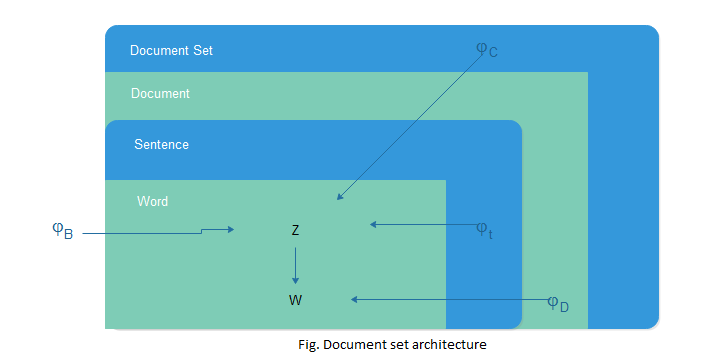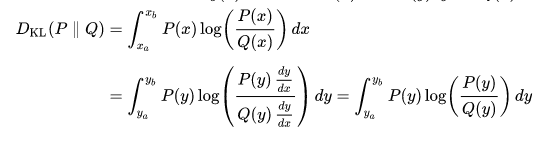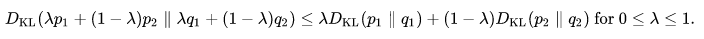×

Search anything:

# KL Sum algorithm for text summarization

#### Machine Learning (ML) Natural Language Processing (NLP)Get this book -> Problems on Array: For Interviews and Competitive Programming

Reading time: 35 minutes | Coding time: 5 minutes

In this article we will discuss K-L sum algorithm (Kullback-Lieber (KL) Sum algorithm) for text summarization which focuses on minimization of summary vocabulary by checking the divergence from the input vocabulary.

It is a sentence selection algorithm where a target length for the summary is fixed (L words). It is a method that greedily adds sentences to a summary so long as it decreases the KL Divergence.

## Objective

The objective of KL sum algorithm is to find a set of sentences whose length is less than L words and the unigram distribution is as similar to the source document.
Unigram distribution - Unigram or n-gram in the field of computational linguistics and probability refers to a contiguous sequence of n items from a given sample of speech or text.

## Analysis

In mathematical statistics, the Kullback-Leiber divergence (relative entropy) is a measure of how one probability distribution is different from another. Less the divergence, more the summary and the document are similar to each other in terms of understandability and meaning conveyed.

## Mathematical analysis

K-L sum algorithm introduces a criterian for selecting a summary S from given document D,

S*=min(KL(PD||PS))
S:[words(S) <=LWhere Ps is the emperical unigram distribution of the candidate summary-S and KL(P||Q) represents Kullback-Leiber(KL) divergence given by:

Σ P(w)log P(w)/Q(w)

This quantity represents the divergence between true distribution P (here document set unigram) and the approximating distribution Q (the summary S distribution).
This summarization method finds a set of summary sentences which closely match the document set unigram distribution.

## Algorithm

It uses greedy optimization approach:

1. Set S={} and d=0
2. while |S|<= L do:
3. for i in [1...ND] , di=KL(Ps||PD)
4. Set S=S + Si with minimum di and d=di
5. Stop if there is no i such that di:<d

The last issue is to set order of the selected sentences. They are ordered according to the value of Pi. The method adopted is to follow the order in the source documents, that is: for each selected sentence Si extracted from document Dj, compute a position index pi (in [0..1]) which reflects the position of Si within Dj. The sentences in the target document are ordered according to the value of pi.

## Computing KL Divergence

KL measures the divergence between a probability distribution P and Q. Think of P as (x1: p1, x2: p2, ..., xn:pn) with Σpi = 1.0 and Q as (y1:q1, ...., yn:qn) with Σqj = 1.0. Assume for now that P and Q are defined over the same outcomes xi - we will discuss the possibility for differences below: Then the definition of KL is:

KL(P,Q) = Σi = 1..n [ pi * log( pi / qi) ]

When we try to compute this formula, we must address two questions:
1.What to do if qi = 0 for some i or pi = 0 for some i?
2.How do we define the formula when P and Q are defined over different samples?

The direct answer is that we always consider that: 0 * log(0) = 0.

### Properties of KL divergence

1. The Kullback–Leibler divergence is always non-negative,
DKL(P||Q)>=0
2. The Kullback–Leibler divergence remains well-defined for continuous distributions, and furthermore is invariant under parameter transformations. For example, if a transformation is made from variable **x** to variable **y(x)**, then, since **P(x)dx=P(y)dy** and **Q(x)dx=Q(y)dy**the Kullback–Leibler divergence may be rewritten:where ya=y(xa) and yb=y(xb).

1. The Kullback–Leibler divergence is additive for independent distributions in much the same way as Shannon entropy.
2. The Kullback–Leibler divergence DKL(P||Q) is convex in the pair of probability mass functions (p,q) i.e. (p1,q1) and (p2,q2) are two pairs of probability mass functions, then## Implementation

We can find necessary tools to implement KL sum method in sumy library in python:

``````pip install sumy
``````

you can install sumy using above command in your terminal.

## Step-wise implementation

``````from sumy._summarizer import AbstractSummarizer
``````

we need to first import AbstractSummarizer from sumy. Then we need to import stopwords , this can be done either using nltk library of python or stop-words library.

``````from nltk.corpus import stopwords
#OR
!pip install stop-words
import stop-words as stop_words
``````

Now , we will define functions to get all the words from a document as well as sentences present in it.

### Pseudocode

``````def _get_all_words_in_doc(self, sentences):
return [w for s in sentences for w in s.words]

def _get_content_words_in_sentence(self, sentence):
normalized_words = self._normalize_words(sentence.words)
normalized_content_words = self._filter_out_stop_words(normalized_words)
return normalized_content_words
``````

Now, in the next step we have to normalize all the words and then remove the stop-words from out sentences.

``````def _normalize_words(self, words):
return [self.normalize_word(w) for w in words]

def _filter_out_stop_words(self, words):
return [w for w in words if w not in self.stop_words]
``````

The step below, we would compute the word frequency and perform tf operations it.

``````def _compute_word_freq(self, list_of_words):
word_freq = {}
for w in list_of_words:
word_freq[w] = word_freq.get(w, 0) + 1
return word_freq

def _get_all_content_words_in_doc(self, sentences):
all_words = self._get_all_words_in_doc(sentences)
content_words = self._filter_out_stop_words(all_words)
normalized_content_words = self._normalize_words(content_words)
return normalized_content_words

def compute_tf(self, sentences):
"""
Computes the normalized term frequency as explained in http://www.tfidf.com/
:type sentences: [sumy.models.dom.Sentence]
"""
content_words = self._get_all_content_words_in_doc(sentences)
content_words_count = len(content_words)
content_words_freq = self._compute_word_freq(content_words)
content_word_tf = dict((w, f / content_words_count) for w, f in content_words_freq.items())
return content_word_tf
``````

Finally, we would calculate the kl divergence

`````` def _kl_divergence(self, summary_freq, doc_freq):
"""
Note: Could import scipy.stats and use scipy.stats.entropy(doc_freq, summary_freq)
but this gives equivalent value without the import
"""
sum_val = 0
for w in summary_freq:
frequency = doc_freq.get(w)
if frequency:  # missing or zero = no frequency
sum_val += frequency * math.log(frequency / summary_freq[w])

return sum_val
``````

This function will return the minimum kl divergence

``````def _find_index_of_best_sentence(self, kls):
"""
the best sentence is the one with the smallest kl_divergence
"""
return kls.index(min(kls))
``````

In the last step, we would generate the summary required, make it a list so that it can be modified

``````        sentences_list = list(sentences)

# get all content words once for efficiency
sentences_as_words = [self._get_content_words_in_sentence(s) for s in sentences]

# Removes one sentence per iteration by adding to summary
while len(sentences_list) > 0:
# will store all the kls values for this pass
kls = []

# converts summary to word list
summary_as_word_list = self._get_all_words_in_doc(summary)

for s in sentences_as_words:
# calculates the joint frequency through combining the word lists
joint_freq = self._joint_freq(s, summary_as_word_list)

# adds the calculated kl divergence to the list in index = sentence used
kls.append(self._kl_divergence(joint_freq, word_freq))

# to consider and then add it into the summary
indexToRemove = self._find_index_of_best_sentence(kls)
best_sentence = sentences_list.pop(indexToRemove)
del sentences_as_words[indexToRemove]
summary.append(best_sentence)
``````

To print the summary

``````print(summary)
``````

## Quick implementation using Sumy library

``````from sumy.parsers.plaintext import PlaintextParser
from sumy.nlp.tokenizers import Tokenizer
from sumy.summarizers.kl import KLSummarizer
``````

It will import all the packages needed for performing summarization.

``````#We Prepare a document with content to be summarized
document = """OpenGenus Foundation is an open-source non-profit organization with the aim to enable people to work offline for a longer stretch, reduce the time spent on searching by exploiting the fact that almost 90% of the searches are same for every generation and to make programming more accessible.
OpenGenus is all about positivity and innovation.
Over 1000 people have contributed to our missions and joined our family. We have been sponsored by three great companies namely Discourse, GitHub and DigitalOcean. We run one of the most popular Internship program and open-source projects and have made a positive impact over people's life.
"""
print(document)
``````

Output

OpenGenus Foundation is an open-source non-profit organization with the aim to enable people to work offline for a longer stretch, reduce the time spent on searching by exploiting the fact that almost 90% of the searches are same for every generation and to make programming more accessible.
OpenGenus is all about positivity and innovation.
Over 1000 people have contributed to our missions and joined our family. We have been sponsored by three great companies namely Discourse, GitHub and DigitalOcean. We run one of the most popular Internship program and open-source projects and have made a positive impact over people's life.

In the next step, we will summarize our document based on the Kl divergence.

``````# For Strings
parser=PlaintextParser.from_string(document,Tokenizer("english"))
# Using KL
summarizer = KLSummarizer()
#Summarize the document with 4 sentences
summary = summarizer(parser.document,4)
``````

It will produce a summary of documents with four sentences in it.

``````#printing the summarized sentences
for sentence in summary:
print(sentence)
``````

Output

OpenGenus is all about positivity and innovation.
Over 1000 people have contributed to our missions and joined our family.
We have been sponsored by three great companies namely Discourse, GitHub and DigitalOcean.
We run one of the most popular Internship program and open-source projects and have made a positive impact over people's life.

Thus we have successfully obtained a summary of out document using KL summarization technique.

## Limitation

Redundancy

Redundancy would only lower the efficiency of algorithm as adding redundant sentences would affect the unigram distribution of words and increase divergence from the source document set.

## Question

#### Which of the following algorithms perform better without stopwords?

KL Sum
SumBasic
LexRank
TF IDF